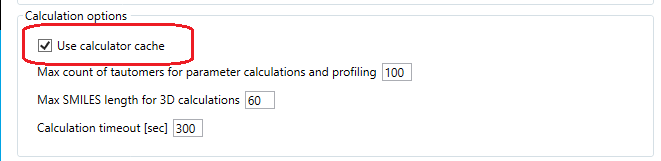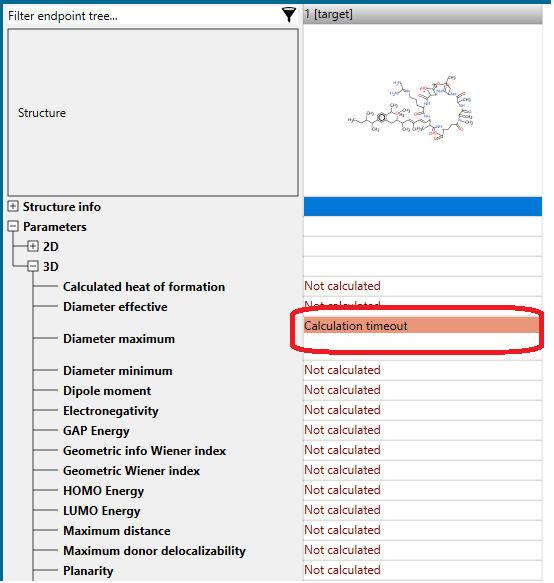General settings allow the user to modify different settings in the program. One of these is Calculation options (Figure 1)Figure 1

The option Use calculator cache (Figure 2) provides the possibility to perform calculation of 2D and 3D parameters with/without using values calculated in advance and stored in the Toolbox cache. The user should be careful when this option is not checked because the process of calculations could be extremely slow in some cases.Figure 2

Max SMILES length for 3D calculations gives the user opportunity to modify that threshold. The default length is set to 60 characters (Figure 3)Figure 3

Calculation timeout defines the time period after which a calculation is halted. The default time is set to 300 seconds (sec) (Figure 4).Figure 4

Once this time finished the "Calculation Timeout" result is displayed on the data matrix (Figure 5).Figure 5

The time could be increased in order to have more time for calculation of parameters (e.g. in case of huge and flexible chemicals; reasonable timeout is 500 000 sec).

Note: Once the settings are modified, please input the target chemical(s) in a new document and run the calculations again.